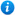* Watermarks do not appear on purchased resources

Preview resource: Click slides to enlarge

# Multiply by Four

KS2 Maths Teaching Resources: Multiply by Four

Year 3 maths programme of study - Number (multiplication and division):

• recall and use multiplication and division facts for the 3, 4 and 8 multiplication tables

In 'Multiply by Four' pupils practise the x 4 multiplication table.

'Multiply by Four' is an animated PowerPoint presentation which includes:

• 2 worksheets
• x 4 flash cards
• interactive activities

'Multiply by Four' is completely editable so that teachers can adapt each slide to suit their individual teaching needs.

To take a closer look at 'Multiply by Four' please click on the PowerPoint images opposite.

Our Price : £1.99 / 2 Credits